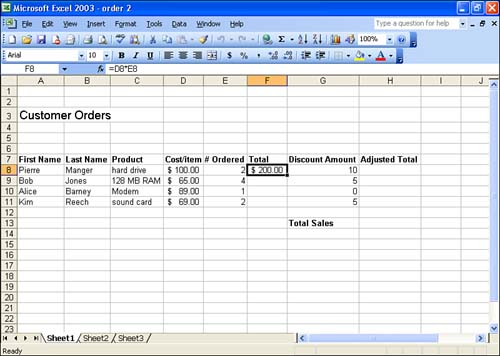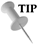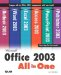# Copying Formulas

Copying labels and values in Excel is no big deal. You can use the Copy and Paste commands (discussed in Lesson 8, "Editing Worksheets") or you can use some of the Fill features discussed in Lesson 2, "Entering Data into the Worksheet." Copying or moving formulas, however, is a little trickier.

Suppose that you create the formula =D8*E8 and place it into cell F8, as shown in Figure 4.1. You have designed the formula to reference cells D8 and E8. However, Excel looks at these cell references a little differently. Excel sees cell D8 as the entry that is located two cells to the left of F8 (where the formula has been placed, in this example). It sees cell E8 as being the location that is one cell to the left of F8.

##### Figure 4.1. Formulas you place in Excel use relative referencing for calculations.Excel's method of referencing cells is called relative referencing . The great thing about this method of referencing cells is that when you copy a formula to a new location, it adjusts to the relative cell references around it and provides you with the correct calculation.

For example, you could copy the formula in cell F8 to cell F9, and Excel would use relative referencing to change the cell addresses in the formula. The original formula =D8*E8 would appear as D9*E9 when copied to cell F9.

Relative referencing is very useful in most situations where you copy a formula to several cells in the same column. However, it can get you into trouble when you cut and paste a formula to a new location or copy the formula to a location where it can no longer reference the appropriate cells to provide you with the correct calculation.

In these situations, you must use absolute referencing so that the formula references only specified cells and does not change the cell references in the formula when pasted to the new location. Absolute referencing is discussed in the next section.

You can copy formulas using the Copy and Paste commands (see Lesson 8); however, when you need to copy a formula to multiple locations, you can also use the Fill feature discussed in Lesson 2.

Because Excel uses relative referencing by default, the formula adjusts to each of its new locations. An alternative way to "copy" a formula to multiple adjacent cells is to select all cells that will contain the formula before you actually write the formula in the first cell. Follow these steps:

1. Select all the cells that will contain the formula (dragging so that the cell that you will write the formula in is the first of the selected group of cells).

2. Enter the formula into the first cell.

3. Press Ctrl+Enter and the formula is placed in all the selected cells.Get an Error? If you get an error message in a cell after copying a formula, verify the cell references in the copied formula. For more information on sorting out cell referencing, see the next section in this lesson.Microsoft Office 2003 All-in-One
ISBN: B005HKSHB2
EAN: N/A
Year: 2002
Pages: 660
Authors: Joe Habraken

Similar book on Amazon

flylib.com © 2008-2017.
If you may any questions please contact us: flylib@qtcs.net